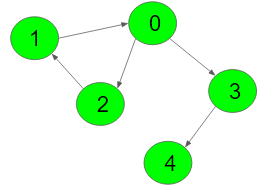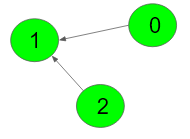Showing:HandleScore
@Ibrahim Nash 6560
@mb1973 5777
@Quandray 5271
@akhayrutdinov 5111
@saiujwal13083 5074
@kirtidee18 4356
@sanjay05 3762
@mantu_singh 3638
@gfgaccount 3601
@marius_valentin_dragoi 3525
Mother Vertex
Easy Accuracy: 49.76% Submissions: 13298 Points: 2

Given a Directed Graph, find a Mother Vertex in the Graph (if present).
A Mother Vertex is a vertex through which we can reach all the other vertices of the Graph.

Example 1:

Input:Output: 0
Explanation: According to the given edges, all
nodes can be reaced from nodes from 0, 1 and 2.
But, since 0 is minimum among 0,1 and 3, so 0
is the output.


Example 2:

Input:Output: -1
Explanation: According to the given edges,
no vertices are there from where we can
reach all vertices. So, output is -1.


You don't need to read or print anything. Your task is to complete the function findMotherVertex() which takes V denoting the number of vertices and adjacency list as imput parameter and returns the verticex from through which we can traverse all other vertices of the graph. If there is more than one possible nodes then returns the node with minimum value.If not possible returns -1.

Expected Time Complexity: O(V + E)
Expected Space Compelxity: O(V)

Constraints:
1 ≤ V ≤ 500

### Editorial

We strongly recommend solving this problem on your own before viewing its editorial. Do you still want to view the editorial?

#### My Submissions: